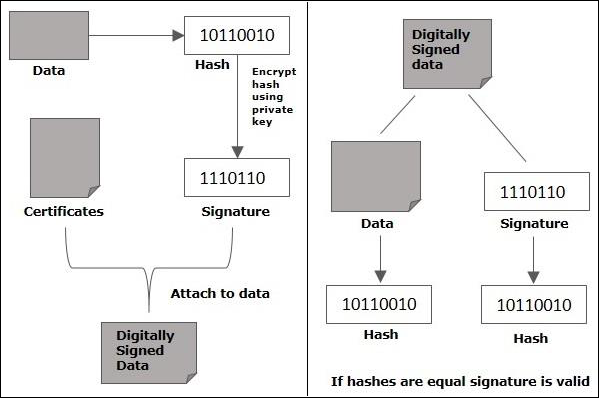# Java Cryptography - Creating Signature

Digital signatures allow us to verify the author, date and time of signatures, authenticate the message contents. It also includes authentication function for additional capabilities.In this section, we will learn about the different reasons that call for the use of digital signature. There are several reasons to implement digital signatures to communications −

### Authentication

Digital signatures help to authenticate the sources of messages. For example, if a bank’s branch office sends a message to central office, requesting for change in balance of an account. If the central office could not authenticate that message is sent from an authorized source, acting of such request could be a grave mistake.

### Integrity

Once the message is signed, any change in the message would invalidate the signature.

### Non-repudiation

By this property, any entity that has signed some information cannot at a later time deny having signed it.

## Creating the digital signature

Let us now learn how to create a digital signature. You can create digital signature using Java following the steps given below.

### Step 1: Create a KeyPairGenerator object

The KeyPairGenerator class provides getInstance() method which accepts a String variable representing the required key-generating algorithm and returns a KeyPairGenerator object that generates keys.

Create KeyPairGenerator object using the getInstance() method as shown below.

```//Creating KeyPair generator object
KeyPairGenerator keyPairGen = KeyPairGenerator.getInstance("DSA");
```

### Step 2: Initialize the KeyPairGenerator object

The KeyPairGenerator class provides a method named initialize() this method is used to initialize the key pair generator. This method accepts an integer value representing the key size.

Initialize the KeyPairGenerator object created in the previous step using the initialize() method as shown below.

```//Initializing the KeyPairGenerator
keyPairGen.initialize(2048);
```

### Step 3: Generate the KeyPairGenerator

You can generate the KeyPair using the generateKeyPair() method. Generate the key pair using the generateKeyPair() method as shown below.

```//Generate the pair of keys
KeyPair pair = keyPairGen.generateKeyPair();
```

### Step 4: Get the private key from the pair

You can get the private key from the generated KeyPair object using the getPrivate() method.

Get the private key using the getPrivate() method as shown below.

```//Getting the private key from the key pair
PrivateKey privKey = pair.getPrivate();
```

### Step 5: Create a signature object

The getInstance() method of the Signature class accepts a string parameter representing required signature algorithm and returns the respective Signature object.

Create an object of the Signature class using the getInstance() method.

```//Creating a Signature object
Signature sign = Signature.getInstance("SHA256withDSA");
```

### Step 6: Initialize the Signature object

The initSign() method of the Signature class accepts a PrivateKey object and initializes the current Signature object.

Initialize the Signature object created in the previous step using the initSign() method as shown below.

```//Initialize the signature
sign.initSign(privKey);
```

### Step 7: Add data to the Signature object

The update() method of the Signature class accepts a byte array representing the data to be signed or verified and updates the current object with the data given.

Update the initialized Signature object by passing the data to be signed to the update() method in the form of byte array as shown below.

```byte[] bytes = "Hello how are you".getBytes();

sign.update(bytes);
```

### Step 8: Calculate the Signature

The sign() method of the Signature class returns the signature bytes of the updated data.

Calculate the Signature using the sign() method as shown below.

```//Calculating the signature
byte[] signature = sign.sign();
```

Example

Following Java program accepts a message from the user and generates a digital signature for the given message.

```import java.security.KeyPair;
import java.security.KeyPairGenerator;
import java.security.PrivateKey;
import java.security.Signature;
import java.util.Scanner;

public class CreatingDigitalSignature {
public static void main(String args[]) throws Exception {
//Accepting text from user
Scanner sc = new Scanner(System.in);
System.out.println("Enter some text");
String msg = sc.nextLine();

//Creating KeyPair generator object
KeyPairGenerator keyPairGen = KeyPairGenerator.getInstance("DSA");

//Initializing the key pair generator
keyPairGen.initialize(2048);

//Generate the pair of keys
KeyPair pair = keyPairGen.generateKeyPair();

//Getting the private key from the key pair
PrivateKey privKey = pair.getPrivate();

//Creating a Signature object
Signature sign = Signature.getInstance("SHA256withDSA");

//Initialize the signature
sign.initSign(privKey);
byte[] bytes = "msg".getBytes();

sign.update(bytes);

//Calculating the signature
byte[] signature = sign.sign();

//Printing the signature
System.out.println("Digital signature for given text: "+new String(signature, "UTF8"));
}
}
```

Output

The above program generates the following output −

```Enter some text
Hi how are you
Digital signature for given text: 0=@gRD???-?.???? /yGL?i??a!?
```